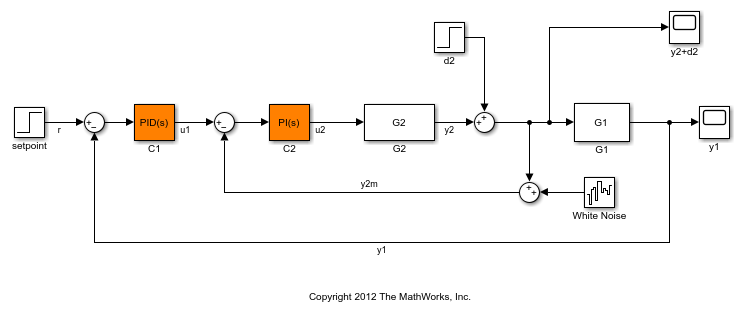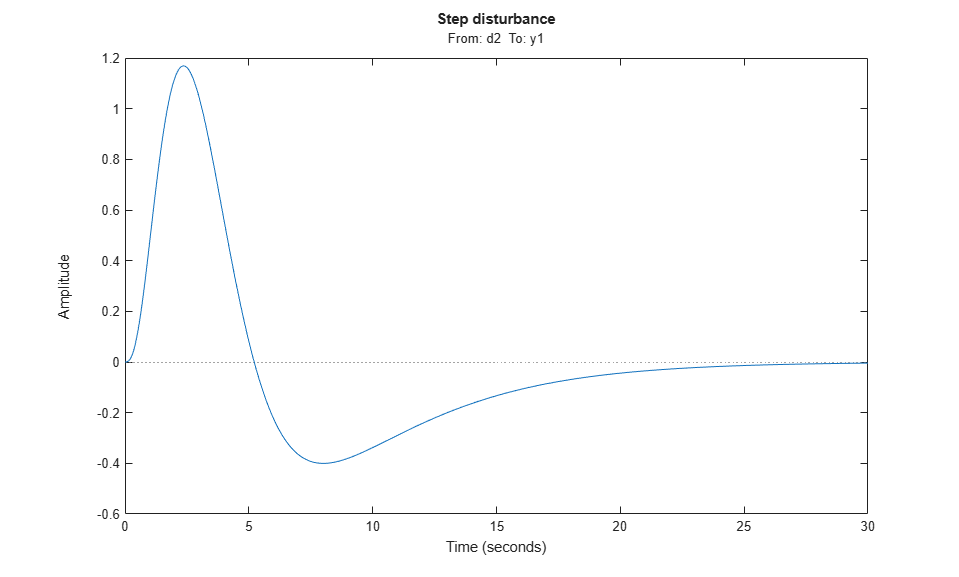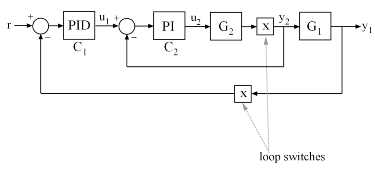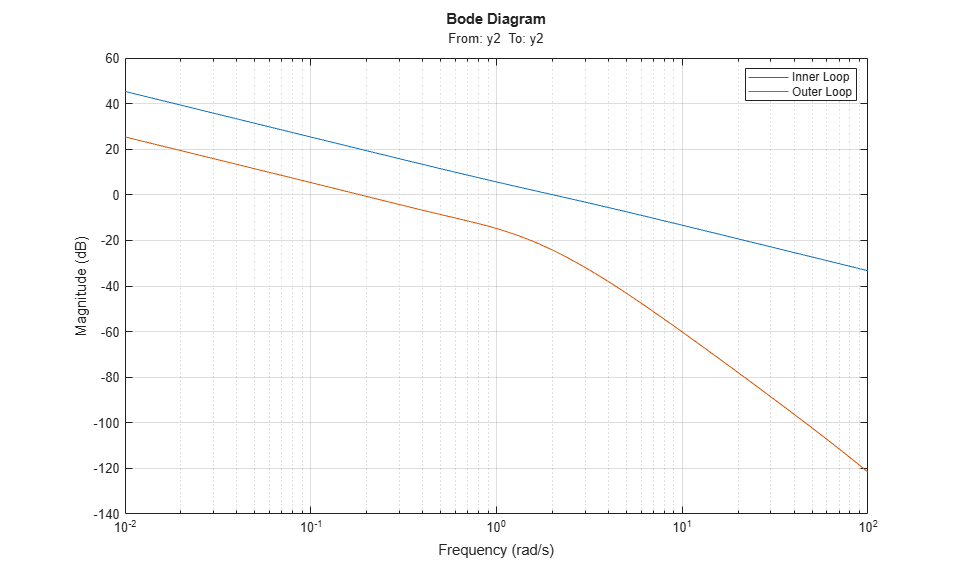Documentation

## Tuning Multiloop Control Systems

This example shows how to jointly tune the inner and outer loops of a cascade architecture with the `systune` command.

### Cascaded PID Loops

Cascade control is often used to achieve smooth tracking with fast disturbance rejection. The simplest cascade architecture involves two control loops (inner and outer) as shown in the block diagram below. The inner loop is typically faster than the outer loop to reject disturbances before they propagate to the outer loop. (Simulink® is not supported in MATLAB® Online.)

```open_system('rct_cascade') ```### Plant Models and Bandwidth Requirements

In this example, the inner loop plant `G2` is

``

and the outer loop plant `G1` is

``
```G2 = zpk([],-2,3); G1 = zpk([],[-1 -1 -1],10); ```

We use a PI controller in the inner loop and a PID controller in the outer loop. The outer loop must have a bandwidth of at least 0.2 rad/s and the inner loop bandwidth should be ten times larger for adequate disturbance rejection.

### Tuning the PID Controllers with SYSTUNE

When the control system is modeled in Simulink, use the `slTuner` interface in Simulink Control Design™ to set up the tuning task. List the tunable blocks, mark the signals `r` and `d2` as inputs of interest, and mark the signals `y1` and `y2` as locations where to measure open-loop transfers and specify loop shapes.

```ST0 = slTuner('rct_cascade',{'C1','C2'}); addPoint(ST0,{'r','d2','y1','y2'}) ```

You can query the current values of `C1` and `C2` in the Simulink model using `showTunable`. The control system is unstable for these initial values as confirmed by simulating the Simulink model.

```showTunable(ST0) ```
```Block 1: rct_cascade/C1 = 1 Kp + Ki * --- s with Kp = 0.1, Ki = 0.1 Name: C1 Continuous-time PI controller in parallel form. ----------------------------------- Block 2: rct_cascade/C2 = 1 Kp + Ki * --- s with Kp = 0.1, Ki = 0.1 Name: C2 Continuous-time PI controller in parallel form. ```

Next use "LoopShape" requirements to specify the desired bandwidths for the inner and outer loops. Useas the target loop shape for the outer loop to enforce integral action with a gain crossover frequency at 0.2 rad/s:

```% Outer loop bandwidth = 0.2 s = tf('s'); Req1 = TuningGoal.LoopShape('y1',0.2/s); % loop transfer measured at y1 Req1.Name = 'Outer Loop'; ```

Usefor the inner loop to make it ten times faster (higher bandwidth) than the outer loop. To constrain the inner loop transfer, make sure to open the outer loop by specifying `y1` as a loop opening:

```% Inner loop bandwidth = 2 Req2 = TuningGoal.LoopShape('y2',2/s); % loop transfer measured at y2 Req2.Openings = 'y1'; % with outer loop opened at y1 Req2.Name = 'Inner Loop'; ```

You can now tune the PID gains in `C1` and `C2` with `systune`:

```ST = systune(ST0,[Req1,Req2]); ```
```Final: Soft = 0.859, Hard = -Inf, Iterations = 67 ```

Use `showTunable` to see the tuned PID gains.

```showTunable(ST) ```
```Block 1: rct_cascade/C1 = 1 s Kp + Ki * --- + Kd * -------- s Tf*s+1 with Kp = 0.0521, Ki = 0.0187, Kd = 0.0477, Tf = 0.00646 Name: C1 Continuous-time PIDF controller in parallel form. ----------------------------------- Block 2: rct_cascade/C2 = 1 Kp + Ki * --- s with Kp = 0.721, Ki = 1.23 Name: C2 Continuous-time PI controller in parallel form. ```

### Validating the Design

The final value is less than 1 which means that `systune` successfully met both loop shape requirements. Confirm this by inspecting the tuned control system `ST` with `viewGoal`

```viewGoal([Req1,Req2],ST) ```Note that the inner and outer loops have the desired gain crossover frequencies. To further validate the design, plot the tuned responses to a step command r and step disturbance d2:

```% Response to a step command H = getIOTransfer(ST,'r','y1'); clf, step(H,30), title('Step command') ``````% Response to a step disturbance H = getIOTransfer(ST,'d2','y1'); step(H,30), title('Step disturbance') ```Once you are satisfied with the linear analysis results, use `writeBlockValue` to write the tuned PID gains back to the Simulink blocks. You can then conduct a more thorough validation in Simulink.

```writeBlockValue(ST) ```

### Equivalent Workflow in MATLAB

If you do not have a Simulink model of the control system, you can perform the same steps using LTI models of the plant and Control Design blocks to model the tunable elements.Figure 1: Cascade Architecture

First create parametric models of the tunable PI and PID controllers.

```C1 = tunablePID('C1','pid'); C2 = tunablePID('C2','pi'); ```

Then use "analysis point" blocks to mark the loop opening locations `y1` and `y2`.

```LS1 = AnalysisPoint('y1'); LS2 = AnalysisPoint('y2'); ```

Finally, create a closed-loop model `T0` of the overall control system by closing each feedback loop. The result is a generalized state-space model depending on the tunable elements `C1` and `C2`.

```InnerCL = feedback(LS2*G2*C2,1); T0 = feedback(G1*InnerCL*C1,LS1); T0.InputName = 'r'; T0.OutputName = 'y1'; ```

You can now tune the PID gains in `C1` and `C2` with `systune`.

```T = systune(T0,[Req1,Req2]); ```
```Final: Soft = 0.86, Hard = -Inf, Iterations = 130 ```

As before, use `getIOTransfer` to compute and plot the tuned responses to a step command r and step disturbance entering at the location `y2`:

```% Response to a step command H = getIOTransfer(T,'r','y1'); clf, step(H,30), title('Step command') ``````% Response to a step disturbance H = getIOTransfer(T,'y2','y1'); step(H,30), title('Step disturbance') ```You can also plot the open-loop gains for the inner and outer loops to validate the bandwidth requirements. Note the -1 sign to compute the negative-feedback open-loop transfer:

```L1 = getLoopTransfer(T,'y1',-1); % crossover should be at .2 L2 = getLoopTransfer(T,'y2',-1,'y1'); % crossover should be at 2 bodemag(L2,L1,{1e-2,1e2}), grid legend('Inner Loop','Outer Loop') ```